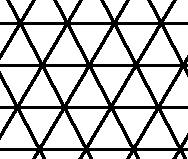# Explain this math term to me

## Recommended Posts

Why, when you are determining the area of a square, is the answer always squared? I mean I'm assuming it's because it's a square, but if you are multiplying out your sides to get area, why would you show the answer as squared? It seems confusing.

I just want to explain it in idiot's terms to ds (not cuz he is an idiot, but because I am). ;-p

##### Share on other sites

When finding the area of any two dimensional object, you use (X units squared) to denote that it is measuring the area of a two dimensional object. So if you are finding volume, you would use (X units cubed) because it is a three dimensional object. If we knew 4 dimensions, similar suit would follow.

Does that make sense? Is that kind of what you were asking?

##### Share on other sites

Think about AREA as being lots of tiny squares of paper. When you find the area of a 2 dimensional figure (square, triangle, rectangle...) you are really finding out how many of those tiny squares will fit inside.

The UNITS (cm, inches, ft...) that you multiplied together to find the area are the size of the tiny squares. If an object has an area of 4 square-inches that tells us that (4) tiny 1-inch by 1-inch squares would fit inside.

If a rectangle had measurements of 5in by 7in then the AREA (formula is L x W) would be 35 square-inches. So 35 1in by 1in squares would be needed to fill up the inside.

Triangles are the same way--but when you picture the tiny squares of area you have to realize that the 'squares' can be pieced together. Using the math formula for the area of a triangle will give you an answer with square units.

______

Now to add more to the fire...

When you are working problems that involve VOLUME you end up with an answer that has cubic units. Instead of 2-dimentional squares you now have 3-dimentional cubes (think square ice cubes). How many square ice cubes will this figure hold? A figure with dimentions L= 5in, W=3in and H=2in will have a total AREA of 30 cubic inches (also written as 30in^3---little 3 is up in the air).

HTH

Jann

##### Share on other sites

Let's say the rectangle is 1 ft by 1 ft so you multiply and get 1 ft, ahh but it is a square foot...a box literally that is 1 ft on each side. You are defining the area enclosed by that shape, in this example a literal square. The same is true for other area problems, so a 3 ft by 10 ft rectangle would be 30 square feet...30 one foot by one foot squares would fit in the space.

The other point is that you always must multiply the units of measurement as well and that is represented by ft^2 or whatever the unit is. Thus volume becomes cubic feet or centimeters. This will be really important later on when you start dealing with physics in particular where you have varying units that have to be multiplied or divided. Oh, I know an easy example of this...miles per hour. rate X time = distance. So let's say you travel 100 miles in 2 hours. The obvious speed is 50 mph... The math is 100 miles divided by 2 hours which reduces to 50 miles/1 hour or 50 mph....making sense?

Hope this helps and you're not an idiot!:D

##### Share on other sites

The above posts are excellent. If you need to explain this to a student, it's nice to illustrate it with a checkerboard. It's got 8 squares across and 8 squares down, and it's 64 square units (chances are each square is a bit larger than an inch by an inch).

##### Share on other sites

What if the lattice points on a graph are not orthogonal? Would we refer to the area of a figure as being "parallelogrammed"?## Join the conversation

You can post now and register later. If you have an account, sign in now to post with your account.×   Pasted as rich text.   Paste as plain text instead

Only 75 emoji are allowed.